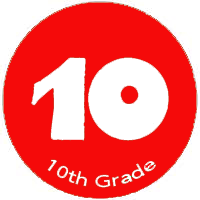Price \$35/Session
Duration 1 Session
Credit Week

Course Description:

The students will receive instruction in and demonstrate the ability to perform  mathematical functions, problem-solving and reasoning and proof in key areas of Algebra,Geometry,Trigonometry,patterns and relationships,Data analysis and probability.

Textbook: Math 10
HIGH SCHOOL MATH COMMON-CORE GEOMETRY PRACTICE/PROBLEM SOLVING WORKBOOK GRADE 9/10 3/15/11 Edition

Materials:

• Student Textbook & homework book
•  Problem of the Day
•  CD / DVD support
•  Practice and Enrichment Worksheets
•  Manipulative materials
•  Calculators
•  Assessment Sourcebook

Time Allotment:
45 minutes per day, 2 days per week

Course Content:

• Algebra review-scale drawings,linear and quadratic equations.
• Points,lines and segments-midpoint and distance formula.
• Parallel and perpendicular lines-identifying and constructing.
• Triangles-classification,Angle-sum theorem.
• Two and three dimensional figures-review,nets ,drawings and cross sections.
• Logic-Hypothesis,conditionals and negations.
• Congruent triangles-SSS,SAS,ASA and AAS theorems.
• Transformations-translation,reflection,rotation and dilation.
• Triangles-midsegments,bisectors and identify medians.
• Symmetry and similarity.
• Circles-arc measure and arc length,tangent lines.
• Vectors-Add and subtract ,find magnitude and component form of vectors.

Areas to be evaluated:

•  Class participation
•  Homework assignments
•  Tests
•  Quizzes
•  Group projects
•  Graphing project
•  Review games and activities